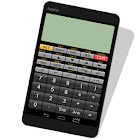Panecal Scientific Calculator

• SEARCH TYPE

All Android applications categories

All Android games categories# Panecal Scientific Calculator

by: 57.5K 8.4

8.4 Users
rating

## Screenshots

Description

Editing and recalculating formulas
Panecal scientific calculator can display and correct mathematical formulas. You can use it to check formulas, which enables you to prevent input mistakes. Panecal has functions such as editing and recalculating formulas entered in the past. You can calculate quickly by using formulas in the past.

A cursor is displayed on the screen, and you can tap the screen or push arrow keys to quickly move to the location you want to edit. Panecal allow you to swipe to scroll through formulas, as well as copying and pasting using long tapping, which makes it a strong and flexible application with an intuitive user interface.

Features
- Arithmetic operations, inverse trigonometric functions, logarithmic functions, power functions, power root functions, factorials, absolute values,degrees-miniutes-seconds and percent calculations
- FloatPt (floating decimal mode), Fix (fixed decimal mode), Sci (index mode), and Eng (index is in multiples of 3) for displaying values
- BIN, OCT, DEC, HEX and n-base number calcutation and conversion (8bits, 16bits, 32bits)
- Degrees-miniutes-seconds conversion
- Calculations with parentheses
- M+ / M- memory and 6 types (A-F) of variable memory
- Constant table
- Units conversion
- Decimal point character and grouping separator settings
- Easy editing by using a cursor operation
- Scroll up/down of formula lines
- Cut and copy and paste text
- Expressions history and answers history table
- Grouping separator and decimal point settings
- Vibration settings
- Portrate and Horizontal screen settngs
- Font size settings

operations
Four arithmetic operations:
1 + 2 - 3 × 4 ÷ 5 = 0.6

Root:
√( 2 × 2 ) = 2

Trigonometric functions:
sin(30) = 0.5
asin(0.5) = 30
(Depends on DRG settings)

Parentheses:
( 1 + 2 ) × ( 3 + 4 ) = 21
( 1 + 2 ) ( 3 + 4 ) ( 5 + 6 ) = 231

Percentages:
500 + 20% = 600
500 - 20% = 400
500 × 20% = 100
100 ÷ 500% = 20

Degree, Minute, Second
30°30 = 30.5
1°30+2°45 = 4°15 = 4.25 (conversion by tapping [°’”] key)

BIN, OCT, DEC, HEX and n-base number conversion
1111(BIN) 0F(HEX) key operation:[CLR][BIN]1111[HEX]

Grouping separator and decimal point
123,456,789.1 + 0.02 = 123,456,789.12
123.456.789,1 + 0,02 = 123.456.789,12
(Depends on settings)

Disclaimer
Please note in advance that Appsys is not responsible for any damage or lost profits caused by the use of this software, or any claims from third parties.

Tags: panecal calculator , panecal scientific , panecalscientificcalculator , software panecal scientific calculator , panecal scientific calculator , panecalplus4.2.3 , panecal 9 video software , panecal scientific calculator softonic , panecal plus 2.3.3

## Users review

from 57.537 reviews

"Great"

8.4